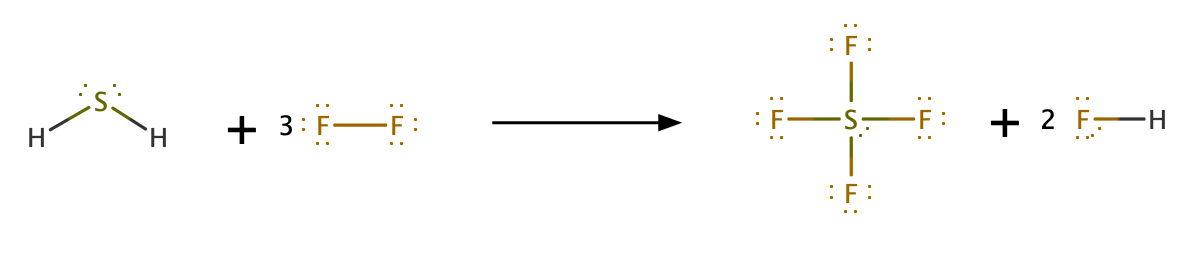# Problem: Use bond energies to predict ΔE for the following reaction:H2S(g) + 3F2 (g) → SF4 (g) + 2HF(g)

###### FREE Expert SolutionReactant:

2 S-H bond: 347 kJ x 2 = 694 kJ

3 F-F bond: 154 kJ x 3 = 462 kJ

Total = 1156 kJ

Product:

4 S-F bond: 327 kJ x 4 = 1308 kJ

2 H-F bond: 565 kJ x 2 = 1130 kJ

Total = 2438 kJ

89% (27 ratings)###### Problem Details

Use bond energies to predict ΔE for the following reaction:

H2S(g) + 3F2 (g) → SF4 (g) + 2HF(g)

Frequently Asked Questions

What scientific concept do you need to know in order to solve this problem?

Our tutors have indicated that to solve this problem you will need to apply the Bond Energy concept. You can view video lessons to learn Bond Energy. Or if you need more Bond Energy practice, you can also practice Bond Energy practice problems.

What professor is this problem relevant for?

Based on our data, we think this problem is relevant for Professor Davis' class at UCF.

What textbook is this problem found in?

Our data indicates that this problem or a close variation was asked in Chemistry: An Atoms First Approach - Zumdahl Atoms 1st 2nd Edition. You can also practice Chemistry: An Atoms First Approach - Zumdahl Atoms 1st 2nd Edition practice problems.